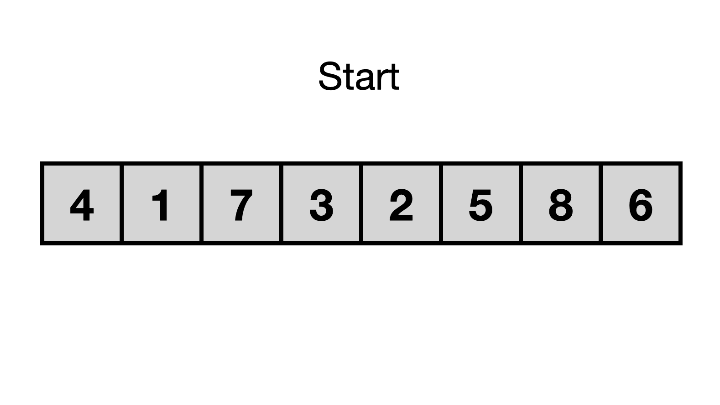# Anan's Sight# Ugh, Not Sorting Algorithms Again!

Before starting the advanced topics for sorting elements, we must have these basic sorting methods:

1. Bubble Sort

2. Quick Sort

3. Merge Sort

4. Insertion Sort

5. Selection Sort

Let's quickly review the algorithm one by one below:

### Bubble Sort

The idea is to place the largest/minimal element in its position and keep doing the same for every other element.

Code:

``````void bubble () {
int n = v.size();
for (int i = 0; i<n; i++) {
for (int j =0; j<n; j++) {
if (v[i] < v[j])
swap(v[i], v[j]);
}
}
}
``````

### Selection SortCode:

``````void selection () {
int n = v.size();
for (int i = 0; i<n; i++) {
for (int j =i+1; j<n; j++) {
if (v[i] > v[j])
swap(v[i], v[j]);
}
}
}
``````

### Insertion SortCode:

``````void insertion () {
int n = v.size();
for(int i=1;i<n;i++){
int x = v[i];
for (int j = i-1; j >=0; j--) {
if (x < v[j])  {
v[j+1] = v[j];
v[j] = x;
}else {
break;
}
}
}
}
``````

### Quick Sort:Code:

``````int partition (vector<int>& v, int low, int high) {
int pivot = v[low];
int i = low +1;
int j = high;
while (true) {
while (i <= j && v[i] <= pivot) {
i++;
}
while ( i <= j && v[j] >= pivot) {
j--;
}
if (i <= j) {
swap (v[i], v[j]);
}else {
break;
}
}
swap(v[low], v[j]);
return j;
}

void quicksort (vector<int>& v, int low, int high)
{
if (low < high) {
int pi = partition (v, low, high);
quicksort(v, low, pi-1);
quicksort(v, pi+1, high);
}
}
``````

### Merge SortCode:

``````#include <bits/stdc++.h>
using namespace std;
int arr[] = {4, 2, 4, 5, -30, -02, 3, -60};
void merge(int low, int mid, int hi)
{
int sa1 = mid - low + 1;
int sa2 = hi - mid;
int L[sa1 + 1], R[sa2 + 1];
for (int i = 0; i < sa1; i++)
{
L[i] = arr[low + i];
}
for (int j = 0; j < sa2; j++)
{
R[j] = arr[mid + 1 + j];
}
L[sa1] = INT_MAX;
R[sa2] = INT_MAX;
int i = 0, j = 0;
for (int k = low; k <= hi; k++)
{
if (L[i] <= R[j])
arr[k] = L[i++];
else
arr[k] = R[j++];
}
}
void mergeSort(int low, int hi)
{
if (low < hi)
{
int mid = (low + hi) / 2;
mergeSort(low, mid);
mergeSort(mid + 1, hi);
merge(low, mid, hi);
}
}
int main()
{
int size = sizeof(arr) / sizeof(arr);
mergeSort(0, size);
for (int i = 0; i < size; i++)
{
cout << arr[i] << " ";
}
}
``````